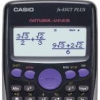Displaying Answer As Fraction On Fx-82Es Plus

4 replies to this topic

#15Pax

5Pax

Newbie

•• Members
•• 1 posts

• Calculators:
Casio fx82ES PLUS

Posted 22 August 2014 - 02:54 PM

Hi! I'm using the fx-82ES PLUS.

When I calculate Pi*cos(Pi/6) the answer I get is 2.720699046. This is equal to (Pi*√3)/2, which is the answer I would like it to show. However, I can't find any way to do thiS. Is this a limitation on the calculator, or is there a setting that changes this?

When i calculate Pi*sin(Pi/6) it gives me (1/2)Pi, so no problem there. Any sugestions?

#2kasio

kasio

Casio Freak

•• Members
•• 161 posts
• Gender:Male

Posted 24 August 2014 - 06:31 PM

Hi! I'm using the fx-82ES PLUS.

When I calculate Pi*cos(Pi/6) the answer I get is 2.720699046. This is equal to (Pi*√3)/2, which is the answer I would like it to show. However, I can't find any way to do thiS. Is this a limitation on the calculator, or is there a setting that changes this?

When i calculate Pi*sin(Pi/6) it gives me (1/2)Pi, so no problem there. Any sugestions?

You can try the S<>D button and make sure you are in MathIO mode (Shift, mode, 1). If that doesn't work, then the calculator cannot display the answer as a fraction

#3pan.gejt

pan.gejt

Casio Freak

•• Members
•• 195 posts
• Gender:Male
• Location:CZ

• Calculators:
.

Posted 25 August 2014 - 07:34 AM

It seems you need CAS calculator.

HP-49G, HP-50G, HP Prime with CAS enabled, Classpad, Ti-89 - all these calcs provide the desired result

HP Prime without CAS enabled, Ti-84C, HP-39G II, fx-82es provide the decimal result

#4kasio

kasio

Casio Freak

•• Members
•• 161 posts
• Gender:Male

Posted 02 September 2014 - 02:40 PM

You can try the S<>D button and make sure you are in MathIO mode (Shift, mode, 1). If that doesn't work, then the calculator cannot display the answer as a fraction

Note that the Casio FX models have little over 100 bytes of working RAM so if the Answer is too large, it cannot show it in the Graphical fraction form.

#5Jan99

Jan99

Newbie

•• Members
•• 3 posts

• Calculators:
Fx-82ES Plus, Fx-991ES Plus, Fx-82AU PLUS II

Posted 29 April 2018 - 03:43 AM

I am also having this problem right now! I've had three casio calculators, and it can all display sin(pi/4) as root2/2 perfectly well. However the new fx-82AU Plus II that I bought just works the opposite and gives me long chains of decimal points which is not what I need and I can't change it to fraction form. Does anyone know any solution?

0 user(s) are reading this topic

0 members, 0 guests, 0 anonymous users